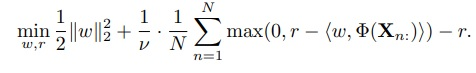# BERT-BiLSTM integration: BiLSTM layer is returning 0 gradient value after first few iterations

Hi,

I have been trying to integrate BERT and BiLSTM as a part of a one class classification experiment and I noticed the gradient values of LSTM are returning 0 after few iteration. I am trying to play with my own defined loss function. Could you please help me finding out the hidden bug in this code? Or is it the usual scenario for such loss function?

This is my model’s structure:

``````class Experiment3(nn.Module):

def __init__(self, bert):
super(Experiment3, self).__init__()
self.bert = BertModel.from_pretrained('bert-base-uncased')
self.lstm = torch.nn.LSTM(768, 128,2,batch_first=True,bidirectional=True)
self.fc = nn.Linear(128*2, 1)
lstm_output, (h,c) = self.lstm(sequence_output[:, 0, :])
weights_=self.fc.weight
weights=(torch.transpose(weights_, 0, 1))
biases=nn.Parameter(torch.tensor([0.01]))
fc_output=torch.matmul(lstm_output,weights)-biases
return weights,biases,fc_output
``````

The sample dataset has 100 data with labels(0 or 1). I have used a dataloader object with random sampling.

``````batch_size=1
train_sampler = RandomSampler(train_data)
``````

The train method is following:

``````def train():
model.train()
batch = [b.to(device) for b in batch]
loss = customLoss(weights,biases,output_)
loss.backward()
for name, param in model.named_parameters():
optimizer.step()

gc.collect()
def customLoss(weight,bias,output):
a=0.5*torch.square(torch.linalg.norm((weight)))
temp=bias-output
z = torch.zeros_like((temp))
b=[torch.mean(torch.maximum(z,temp))]
l2 = a+(1/(0.01))*b-bias
return l2
``````

The driver’s code is following:

``````model = Experiment3(bert)
model = model.to(device)
epochs = 1
current = 1
while current <= epochs:
print(f'\nEpoch {current} / {epochs}:')
train()
current = current + 1
``````

I would appreciate if you help me understanding this NN and its tricky gradient nature.

In `Experiment3.forward` you are recreating the `biases` parameter so note that it won’t be trained and I assume that’s on purpose.

I don’t fully understand your custom loss function. It seems you are adding weight decay to the `b` tensor. If `temp` has a negative value (i.e. if `output>0.01`) your model would create zero gradients, as `torch.maximum` would select `z` and would not backpropagate through the `output` to the model. (Please correct me if I’m missing something)

Also,

sounds strange, since the model would just try to learn the single class. Wouldn’t `return 0` be a perfect classifier in this case?

ptrblck Hi

Thanks for your reply. According to your query on the loss function, I have tried to implement the following loss function:In my code, I extract the `w` from FC layer and set a random value to `bias`. Here I assume the `r=bias`. What do you think on my loss function’s implementation? Am I on the right track?

I have noted your observation regarding the `temp` value. I have increased the value of the `bias` to make the `temp` value positive which in turn shows the `lstm` layers returning the gradient values other than zero.

Here my intention is `w` and `bias` must be trainable parameters. In my implementation, I found FC layer’s `bias` parameter is not learning(it returns None).

Regarding your last query, my intention is just to learn the features of only a single class.

Yes, that’s expected as mentioned in my previous post:

If you want to reuse the `bias` from the linear layer and train it, you should access it and not create a new `nn.Parameter`:

``````bias = self.fc.bias
# use bias
``````

I don’t know if and how it would work. Assuming your model indeed tries to predict a single class, the model would only need to make sure to predict the largest logits for the single class which would not depend of any inputs, so I’m not sure how much the model would “learn” the dataset.

1 Like

Hi,

I have experimented the model with 2 cases:
Case 1: `bias=self.fc.bias`
In this case, I notice either the gradient value of lstm layers’ are showing `0` for each batch(if the bias value is negative) or it starts to show `0`(if the bias value is positive) after certain iterations.
Case 2: a big value is initialized to bias such as `biases=nn.Parameter(torch.tensor())`
In such scenario, I observed the gradient of the FC layers bias is returning a constant value in each iteration, `fc.bias tensor([199.])`.

What might be the reasons behind such behavior?